Outside of the comment stream, I had a surprising amount of people tell me they found my Overland Encounter Article useful (Three: which is three higher than usual). Universally however, the part of it they mentioned was the final paragraph and illustration:

A brief note on die choice: With a single die, all outcomes are equally likely. The more dice you use, the greater central tendency of your roll, and the rarer the high and low values. Using dice of unequal size on the same roll will create a small “plateau” of probabilities in the center. It’s in no way essential to sit and crunch numbers, but keep in mind that if you use 5d6 for your table, the probability of rolling a 5 or a 30 is .01% so don’t expect to ever see it.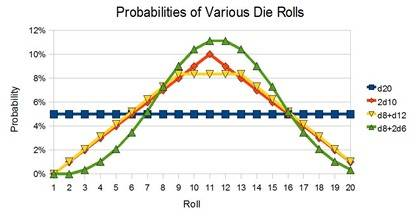Thus, I figured an article going a little more in depth on this particular aspect of die probabilities was in order.  To explore these probabilities, We’ll go through an example:  finding the probabilities for a 1d12+1d6+1d4 roll.  The easiest way to find these probabilities is to make a map of which rolls can create which results. (That’s a boldfaced lie. This is the easiest way.)  To do so, you start with your largest die, and write down all the possible results.  Underneath, those, you write the number of rolls that create each result.  Since we’re still dealing with a single die, those numbers should all be 1 (unless you’re using an oddball die like old-school d20s with 2 numbers each from 1 to 10).

Underneath, write down the possible roll outcomes from your two largest dice.  This is usually from 2 to the sum of their max rolls.  For our example roll, this is a d12 + d6, so our range is 2 to 18. The result should look something like this: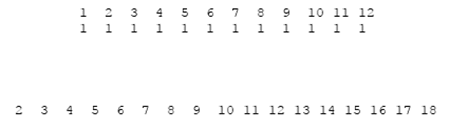Now, from here, we look at each of the results in the d12 + d6 row and map it to a number of results in the above rows. We can only get a 2 on a d12 + d6 roll if we roll a 1 on the d12.  We can get a 3 on the d12 + d6 roll, if the 12 was a 1 or a 2. We can get a 4 from a 1 2 or 3, and we can get a 5 from 1-4.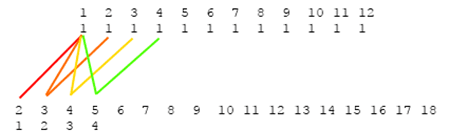Underneath the row of d12 + d6 outcomes, make a note of how many of the d12 outcomes map to each one. What you’re really doing is mapping the number of possible die rolls.  ex: for a d12 + d6 roll of 5, we could start with a d12 roll of 1-4 Since there is only one way to roll a 1, 2, 3, or 4 on a d12, there are four possible rolls on a d12 + d6 that add to 5: 1+4 2+3 3+2 4+1. In this stage, the only time that distinction matters is if your first die doesn’t have one and one way only to roll each of it’s outcomes.  When we finish the mapping, we have a chart that looks like this: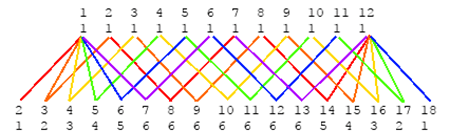Note that our number of outcomes in the bottom row are symmetrical, and they sum to 12 * 6 = 72.  This should always be the case.  And because we’re only dealing with two dice, they follow a predictable pattern: starting at 1, they count up, till they reach the size of your smaller die, plateau at that number for a while, then count back down to 1 at the far end.  The plateau is from 7 (smaller die size +1) to 13 (larger die size +1).

If we were interested in the probabilities of a d12 + d6 roll, we’d have everything we needed right now.  For any given roll, we’ve mapped the number of outcomes that give us that roll, and we know our total number of outcomes.  Since every outcome is equally likely, the probability of any given roll is given by (# of outcomes that give that roll) / (total number of outcomes). Thus the probability of rolling a 4 (or a 16) is 3/72 = 1/24 ~ 4%.  The probability of rolling any result on the plateau (7-13) is 6/72 = 1/12 ~ 8%

To finish our exercise, we add another row of numbers on the bottom with the results of our d12+d6+d4 roll, and map in the same way as before.  The only difference is that the possible outcomes we’re mapping from are no longer all 1, so instead of simply counting the number of outcomes that map, we have to sum the number of outcomes for each result that maps.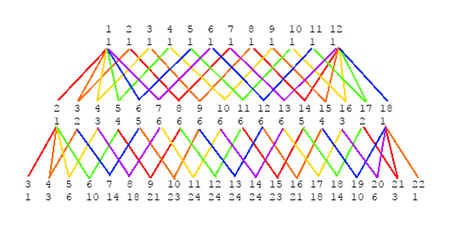So, for example, there are 14 outcomes that can give us a final result of 7 because a result of 3, 4, 5, or 6 on our 1d12+1d6 roll can give us a 7 on our 1d12+1d6+1d4 roll, and on that d12+d6 roll there are 2 ways to get a 3, 3 ways to get a 4, 4 ways to get a 5 and 5 ways to get a 6.  2+3+4+5 = 14.

Like the earlier map, this one follows a few rules.  It always ascends, then descends, it’s always symmetrical, it may have a plateau of size (previous plateau size – new die size + 1), the total outcomes sum to the joint product of all die sizes rolled (12 * 6 * 4 = 288),   and it’s noticeably more bell-curved.

Once again, the formula to find the probability of rolling any given number is (# of outcomes that give that roll) / (total number of outcomes).  For any number on our new plateau, the probability is 24/288 = 1/12 ~ 8%.